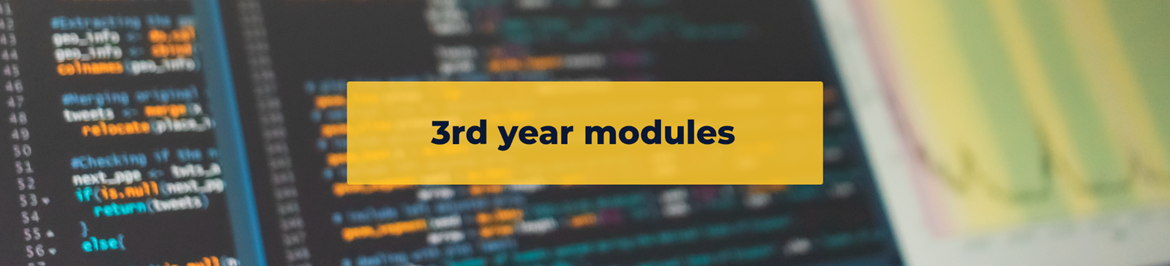# Mathematical Statistics 3

This course consists of the five modules STAS301, STAS321, (STAS322 or STAS302), STAS312, STAS342 which must be passed separately.### Statistics 3

This course consists of the six modules STAS311, STAS331, STAS321, STAS302, STAS312, and STAS342 which must be passed separately.

STAS301: Statistical Inference (24 Credits)

This module consists of 66 single lectures and 28 tutorials during the first semester.

Pre-requisites

A previous pass in STAS201 and a pass or concurrent registration for either MAPM201 - 204 or MATH201 - 204.

Content

Methods of finding estimators’. Criteria for evaluating estimators. Sufficiency and completeness.

1. Confidence intervals: pivotal quantity method and general method.

2. Theory of hypothesis testing: tests for Normal distribution, Binomial and Poisson tests, UMP tests, Generalized likelihood ratio test.### STAS311: Non-Parametric Statistical Procedures (10 Credits)

This module consists of 28 single lectures, 10 practicals and 10 tutorials during the first semester.

Pre-requisites

A previous pass in STAS202.

Content

1. Inferential Single Sample Tests: Binomial Sign Ttest. Wilcoxon Signed-Rank Test. Kolmogorov-Smirnov Goodness of Fit. Randomness Tests.

2. Inferential Two Independent Sample Tests: Mann-Whitney U Test. Kolmogorov-Smirnov Independence Test.

3. Siegel Tukey and Moses Test of Variability. : Contingency tables, 2*2 and r*c. Median test. Wilcoxon Matched Pairs Signed Rank Tests. The McNemar Test.

4. Inferential Tests For More Than Two Independent Samples: Bonferroni-Dunn, Newman-Keuls, Dunnet Tests. Kruskal-Wallis ANOVA.

5. Inferential Tests For More Than Two Dependent Samples: Bonferroni-Dunn, Tukey’s HSD, Friedman ANOVA.

6. Measures of Association: Spearman’s Rank-Order Correlation Coefficient, Goodman and Kruskal’s Gamma.### STAS331: Econometric Models (14 Credits)

This module consists of 38 single lectures, 14 practicals and 14 tutorials during the first semester.

Pre-requisites

A previous pass in STAS202.

Content

1. Single-equation econometric models: Specification, estimation and testing. Distributed Lag Models. Koyck Lag Models.

2. Multi-equation econometric models: Specification, Identification and Estimation: The Hausman test for simultaneity and OLS, 2SLS, 3SLS, FIML and SUR Estimation. Introduction to simulation models.### STAS321: Special Topics in Statistics (6 Credits)

This module consists of 18 single lectures and 12 tutorials during the first semester.

Pre-requisites

A previous pass in one of STAS201 or STAS211

Content

Sample CDF and it’s use for estimation. Order statistics. Sample median, sample range. Tests for goodness of fit: Pearson’s test, Kolmogorov-Smirnov test. Bayesian statistical analysis. Prior and posterior distributions. Conjugate families of distributions. Loss function. Bayes estimators. Bayesian test procedures. Bayesian confidence intervals.### STAS322: Theory of Linear Models (10 Credits)

This module consists of 28 single lectures, 10 practicals and 10 tutorials during the second semester.

Pre-requisites

A previous pass in one of STAS201 or STAS211 and concurrent registration or a previous pass in both MATH203 - 204.

Content

Generalized inverse matrix, elementary matrix, projection matrix, general linear model (LM), least square estimation, maximum likelihood estimation, estimable functions, Gauss-Markov theorem, Statistical methods based on LM: linear regression, polynomial regression, categorical data regression, one way Analysis of Variance (ANOVA), two-way ANOVA, paired comparisons model.### STAS302: Experimental Design and ANOVA (10 credits)

This module consists of 28 single lectures, 10 practicals and 10 tutorials during the second semester.

Pre-requisites

A previous pass in STAS202

Content

1. Basic concepts: experimental unit, factors, levels and treatments.

2. Designs: Completely randomized design. Randomized block design. Factorial designs. ANOVA of completely randomized, randomized block, and factorial experiments. The regression approach for analyzing data generated by experimental designs. Multiple comparisons of treatment means.### STAS312: Time Series Analysis (10 Credits)

This module consists of 28 single lectures, 10 practicals and 10 tutorials during the second semester.

Pre-requisites

A previous pass in STAS202.

Content

Properties of stochastic time series models. Extrapolation and Smoothing. Forecasting: Moving averages, exponential smoothing and the Holt-Winters model, regression models and autoregressive error models. The Box-Jenkins approach: ARMA and ARIMA models.### STAS342: Operations Research (10 Credits)

This module consists of 28 single lectures, 10 practicals and 10 tutorials during the second semester.

Pre-requisites

A previous pass in one of STAS201 or STAS211

Content

OR objectives and models. Linear programming, canonical and standard form. Structure of solution space, extreme points. Basic and non-basic variables, simplex algorithm and simplex table. Two-phase technique. Degeneracy. Transportation technique. Assignment problem, Hungarian method. Basics of Duality theory.